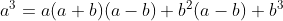# Mahavira: A Mathematical Prodigy of the Ancient India

Mahavira or Mahaviracharya was a 9th century (about 800-870 CE) Jain mathematician, who made significant contributions to the development of Algebra, born in the present-day city of Gulbarga, Karnataka, in southern India. He perhaps took his name to honour the great Jainism reformer Mahavira. He was the author of the earliest Indian text Ganitsarasangraha (dated 850 CE) devoted entirely to mathematics. He was patronised by the king Amoghavarsha of the Rashtrakuta Dynasty. Mahavira stresses the importance of mathematics in both secular and religious life and in all kinds of disciplines including love and cooking. He was the first one to separate Astrology from Mathematics. He is highly respected among Indian Mathematicians because of his establishment of terminology for concepts such as equilateral triangle, isosceles triangle,rhombus, circle and semicircle and the rest of mathematical procedures such as basic operations, reduction of fractions, miscellaneous problems involving a linear or quadratic equation with one unknown, the rule of three (involving proportionality), mixture problems, geometric computations with plane figures, ditches (solids) and shadows. He also included rules for permutations and combinations and for the area of a conch like plane figure (two unequal semi circles stuck together along their diameters).

Mahavira discovered algebraic identities: an identity is an equality type mathematical expression containing some variables, satisfied for any value of the variables.
e.gMahavira in his ‘Ganitsarasangraha’ has given the general formula for combination: in Mathematics, Combination (nCr) is a selection of items from a collection. The formula is as follows:

His book, ‘Ganitsarasangraha’ contains rules for adding fractions of unequal denominators by finding niruddha or L.C.M (least common multiple).

This book gives the rule for area and circumference of Ayatavritta (elongated circle, similar to ellipse), which is one of the most complicated calculations of mathematics. According to him, area of an ellipse is √(πa^2×πb^2 )=πab and the circumference is 2√((6b^2+4a^2)), where a is the major axis and b is the minor axis. Though this formula for circumference does not give accurate value but it has too much importance. Later many Mathematicians gave many formulae for finding the circumference, but only Ramanujan’s formula using calculus gives the most accurate value.

He enumerated six types of fractions and the use of unit fractions is one of the unique contributions of Mahavira and is illustrated in his book. He also found methods to calculate the square root of a number and cube root of a number. He asserted that the square root of a negative number did not exist because it is not a square root of any real number.